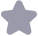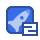### 抢答器程序收藏 2

void main() { TMOD = 0x01; EA = 1; ET0 = 1; TH0 =(65536-9216)/256; TH0 =(65536-9216)/256; while(1) { keying(); if(r == 0) { display(10); } if(d == 5) { TR0 = 1; //开启计时 display(a); r = 1; i = 1; } if(a >= 0 && a <= 10) //倒计时中 { if(d ==1 ||

...展开详情
抢沙发
一个资源只可评论一次，评论内容不能少于5个字
橙子渣渣•签到新秀

累计签到获取，不积跬步，无以至千里，继续坚持！
关注 私信 TA的资源
上传资源赚积分,得勋章
最新推荐